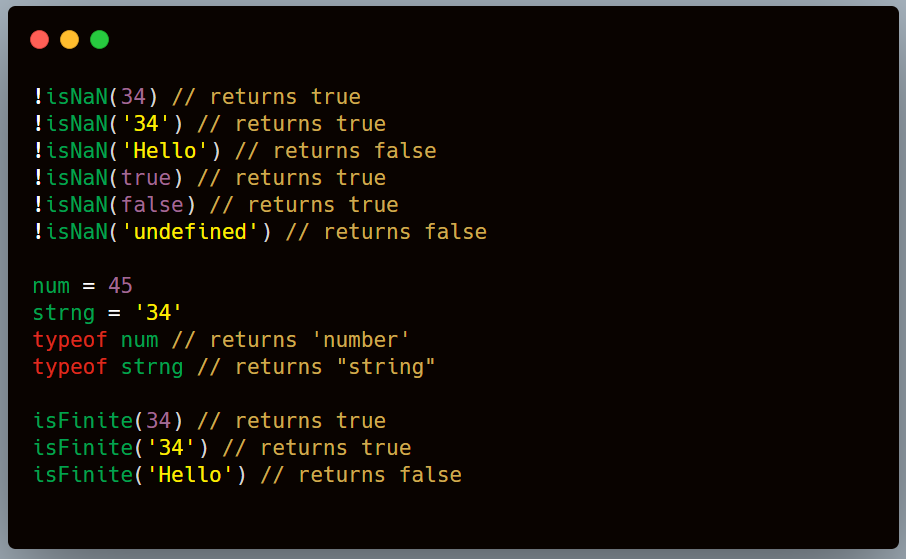# How To Check If A Variable Is A Number In JavaScript

## Here are 3 ways that you can check

I was building a form the other day in Vue and I had to write number validation for the field so had to write the logic to check whether the input value is number or not. I wanted to list out the few ways I learnt which might be helpful to others.Note: In JavaScript, special values like `*NaN*``*Infinity*` and `*-Infinity*` are numbers too - though, we'll be ignoring those values.

### 1. Using isNan()

The `[isNaN()](https://developer.mozilla.org/en-US/docs/Web/JavaScript/Reference/Global_Objects/isNaN)` determines whether a value is `[NaN](https://developer.mozilla.org/en-US/docs/Web/JavaScript/Reference/Global_Objects/NaN)` or not. We can take advantage of this to determine whether a variable is number type or not.

``````var numberOfpushUpsToday = 34;

if (!isNaN(numberOfpushUpsToday)) {
console.log("It is a number");
} else {
console.log("It is not a number");
}
``````
``````!isNaN(34)
// returns true!isNaN('34')
// returns true!isNaN('Hello')
// returns false!isNaN(true)
// returns true
!isNaN(false)
// returns true !isNaN('undefined')
// returns false
``````

Limitations:

1. It returns `true` for the Boolean values because the Boolean values are converted to numbers 0 and 1 accordingly so it would be misleading sometimes.
2. We have same issue for the `null` value as well. It returns true and hence one has to be careful while writing the code.

### 2. Using typeof()

The `[typeof](https://developer.mozilla.org/en-US/docs/Web/JavaScript/Reference/Operators/typeof)` operator returns a string indicating the type of the unevaluated operand.

``````num = 45
strng = '34'typeof num // returns 'number'
typeof strng // returns "string"
typeof undefined // returns "undefined"
typeof null // returns "object"
``````

If the variable is type number, it would return the string `'number'`. We can use this to determine if the variable is number type.

``````var numberOfpushUpsToday = 34;

if (typeof numberOfpushUpsToday === "number") {
console.log("It is a number");
} else {
console.log("It is not a number");
}
``````

The `typeof()` performs much better than `isNaN()`. It correctly determines that a string variable, `null` and Boolean values are not numbers.

### 3. Using Number.isFinite()

The function `[isFinite()](https://developer.mozilla.org/ja/docs/Web/JavaScript/Reference/Global_Objects/isFinite)`determines if the passed value is finite. The arguments are first converted to numbers and then checks if the value is finite. This method works fine with undefined and Boolean contrary to others and hence this is the most best way among all the methods mentioned above.

``````Number.isFinite(34);
// returns trueNumber.isFinite('Hello')
// returns falseNumber.isFinite(undefined)
// returns falseNumber.isFinite(true)
// returns falseNumber.isFinite(null)
// returns false
``````
``````var numberOfpushUpsToday = 34;

if (Number.isFinite(numberOfpushUpsToday)) {
console.log("It is a number");
} else {
console.log("It is not a number");
}
``````

### Conclusion

Although these methods can get tricky with Boolean values, undefined and null, they are helpful in solving some problems in day to day life. One just have to be careful while writing the code according to their needs.